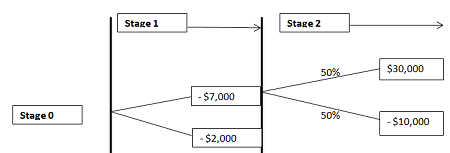# At Stage 2 of the decision tree it shows that if a project is successful, the payoff will be...

## Question:

At Stage 2 of the decision tree it shows that if a project is successful, the payoff will be $30,000 with a 50% chance of occurrence. There is also the 50% chance of a -$10,000 payoff.

The cost of getting to Stage 2 1 year out is $5,000. However, in the Stage 1, the project is losing money, which is$2,000.

You can choose to invest more $5,000 to get in Stage 2 or exit with$2,000 loss.

The cost of capital is 15 percent. Should you continue or not? Why?

## Decision Tree:

In net present value analysis, decision tree approach is a schematic representation of the NPV derived as a result of multiple decisions and possible outcomes against each decisions (with probabilities in most cases ), at each stage of a multistage project. Finally the highest NPV is selected to choose the most acceptable alternative.

The key limitation of the analysis is that the outcomes must be discrete , and probabilities of the outcomes and its effect on cash flows should be estimated at the initial stage.

We draw the decision tree as below:Cost of capital = 15%

Expected cash flow at stage 2 = $30,000*50% + (-$10,000)*50% = $10,000 Present Value of the stage 2 cash flow at stage 1 =$ 10,000/1.15 = $8,696 But net expense at stage 1 to proceed for stare 2 =$7,000

So, net expected cash flow at year 1 = $8,696 -$7,000 =$1,696 So, the present value of the cash flow =$ 1,696/1.15 = $1,475 ............................(1) But considering the loss absorption and exit at stage 1 net cash flow = -$2,000

PV of the cash flow = - $2,000/1.15 = -$ 1,739 ........................................................(2)

From (1) and (2) we conclude that the project should be continued at stage (2) as PV of cash flow (1) > PV of cash flow (2)